Difference equation

(diff) ← Older revision | Latest revision (diff) | Newer revision → (diff)

An equation containing finite differences of an unknown function. Letbe a function depending on an integer argument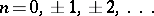; let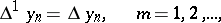be the finite differences. The expression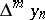contains values of the functionat thepoints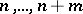. The formula(1)

is valid. An equation of the form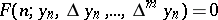(2)

is called a difference equation, whereis an unknown andis a given function. Replacing the finite differences in (2) by their expressions in the values of the desired function according to (1), it reduces to an equation of the form(3)

If,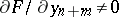, that is, if equation (3) really does contain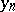as well as, then equation (3) is called an-th order difference equation.

The most developed theory is that of linear difference equations, which has much in common with the theory of linear ordinary differential equations (see ). An equation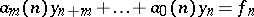(4)

is an-th order linear difference equation. Here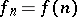is a given function and the,, are given coefficients,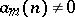,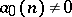. Every function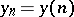satisfying equation (4) is called a solution to the difference equation. As in the case of differential equations one distinguishes particular and general solutions of the difference equation (4). A general solution to the difference equation (4) is a solution, depending onarbitrary parameters, such that each particular solution can be obtained from it by giving a certain value to the parameters. Usually the actual values of the parameters are found from supplementary conditions. The Cauchy problem is typical: Given, find the solutionto equation (4) when. The existence of and a method for constructing a solution to the difference equation (4) are established according to the following scheme. Along with (4) the homogeneous difference equation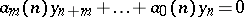(5)

is considered.

The following assertions are true:

1) Let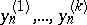be solutions to equation (5) and let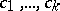be an arbitrary collection of constants. Then the function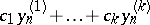is also a solution to equation (5).

2) If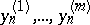aresolutions to equation (5) and if the determinant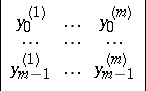is non-zero, then the general solution to the homogeneous difference equation (5) has the form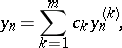(6)

where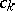are arbitrary constants.

3) The general solution to the non-homogeneous difference equation (4) is the sum of any one of its particular solutions and the general solution of the homogeneous difference equation (5).

A particular solution to the non-homogeneous equation (5) can be constructed by starting from the general solution (6) of the homogeneous equation by the method of variation of parameters (see, for example, ). In the case of a difference equation with constant coefficients,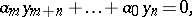(7)

one can findlinearly independent particular solutions immediately. Namely, consider the characteristic equation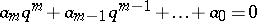(8)

and find its roots. If all the roots are simple, then the functions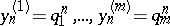are a linearly independent system of solutions to equation (7). When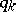is a root of multiplicity, the solutions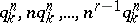are linearly independent.

If the coefficientsare real and equation (8) has a complex root, for example a simple root, then instead of the complex solutionsone obtains two linearly independent real solutions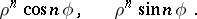Suppose one has a second-order difference equation with constant real coefficients,(9)

The characteristic equationhas the roots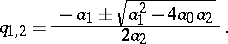Whenit is convenient to write the general solution to (9) in the form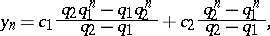(10)

whereand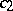are arbitrary constants. If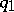and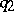are complex conjugate roots:then another representation of the general solution is(11)

In the case of a multiple root the general solution can be obtained by taking limits in (10) or (11). It will have the form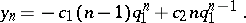One can consider the Cauchy problem or various boundary value problems for second-order difference equations in the same way as for equations of arbitrary order. For example, for the Cauchy problem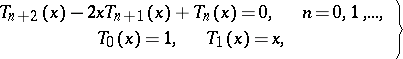(12)

whereis any real number, the solution of (12) is a polynomialof degree(a Chebyshev polynomial of the first kind), defined by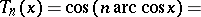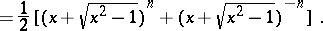A boundary value problem for a second-order difference equation is to find a functionsatisfying, when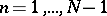, an equation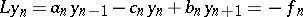(13)

and two linearly independent boundary conditions. Such boundary conditions can be, for example,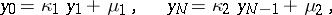(14)

or(15)

The following maximum principle is valid for a second-order difference equation. Given the problem (13), (15), let the conditions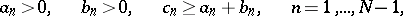be fulfilled. Now if,, then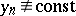cannot have a greatest positive (smallest negative) value when. The maximum principle implies that the boundary value problem (13), (15) is uniquely solvable and that its solution is stable under a change of the boundary conditions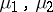and the right-hand side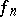. The shooting method (see ) can be applied to solve difference boundary value problems (13), (14).

One has constructed an explicit form of the solution to a non-linear difference equation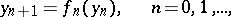(16)

only in isolated, very special cases. For equations of the type (16) one studies qualitative questions on the behaviour of the solutions as, and a stability theory, which on the whole is analogous to the stability theory for ordinary differential equations, has been developed (see , ).

Multi-dimensional difference equations arise for difference approximations to partial differential equations (see , ). For example, the Poisson equationcan be approximated by the difference equation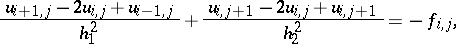where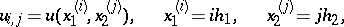and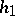and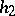are the steps of the grid.

A system of multi-dimensional difference equations together with additional initial and boundary conditions forms a difference scheme. Such questions as the correctness of difference problems, methods for solving them, convergence under grid refinement to the solutions of the original differential equations, are all studied in connection with multi-dimensional difference equations (see Difference schemes, theory of).

Although various mathematical and technical models leading to difference equations exist (see, for example, , ) the basic field of their application is in approximation methods for solving differential equations (see  and ).